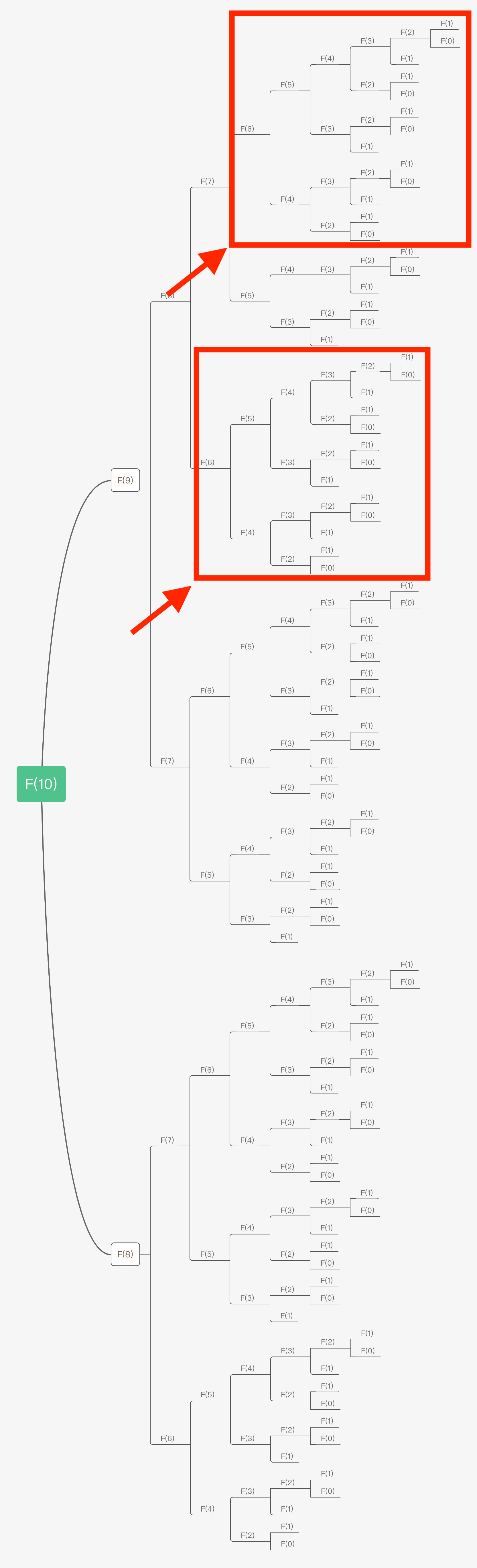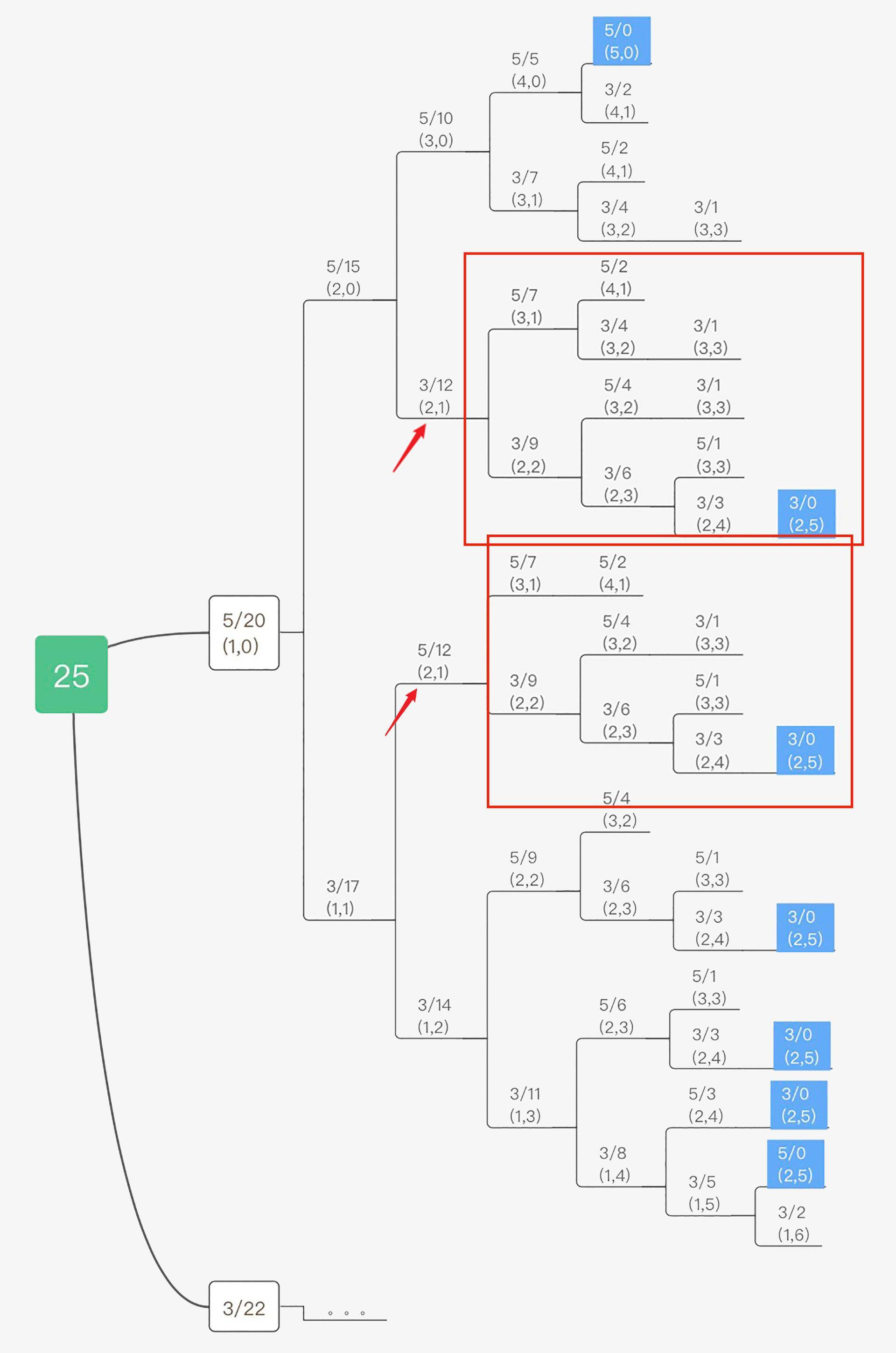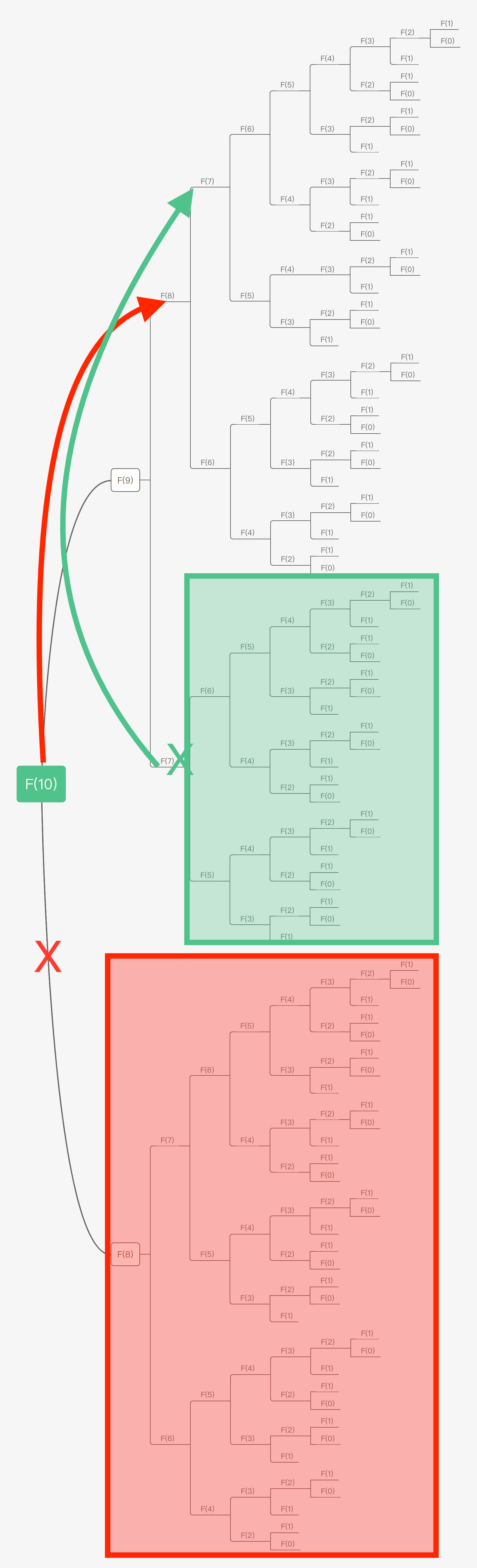03 备忘录：如何避免递归中的重复计算？

## 什么是重叠子问题？

$F(n)=\\left\\{\\begin{array}{c}- 0,n=0\\\\\\- 1,n=1\\\\\\- F(n-1)+F(n-2),n>1- \\end{array}\\right.$

int Fibonacci(int n) {
if (0 == n || 1 == n) { return n; }
if(n > 1) { return Fibonacci(n - 1) + Fibonacci(n - 2); }

return 0; // 如果输入n有误，则返回默认值
}1. 先考虑子问题的个数（即上图中节点的总数），二叉树节点总数为指数级别，因此子问题个数为指数级 O(2n)；
2. 再考虑求解一个子问题的复杂度：没有计算，因此是 O(1)；
3. 综上所述，该算法的时间复杂度是 O(2n)。

## 递归中的备忘录：解决重复计算的法宝

### 备忘录即正义

1. 数组（Array），通常对于简单的问题来说，使用一维数组就足够了。在后续的课程中，你会看到更为复杂的状态存储过程，届时我会指导你使用更高维度（二维甚至三维）的数组来存储状态。
2. 哈希表（Hash table），如果你存储的状态不能直接通过索引找到需要的值（比如斐波那契数列问题，你就可以直接通过数组的索引确定其对应子问题的解是否存在，如果存在你就拿出来直接使用），比如你使用了更高级的数据结构而非简单的数字索引，那么你还可以考虑使用哈希表，即字典来存储中间状态，来避免重复计算的问题。

Java 实现：

int fibonacci(int n, int[] memo) {
if (0 == n || 1 == n) { return n; }
if (memo[n] != 0) { return memo[n]; } // 看来备忘录中找到了之前计算的结果，既然找到了，直接返回，避免重复计算

if(n > 1) {
memo[n] = fibonacci(n - 1, memo) + fibonacci(n - 2, memo);
return memo[n];
}

return 0; // 如果数值无效(比如 < 0)，则返回0
}

int[] memo = new int[n + 1];
return fibonacci(n, memo);
}


C++ 实现：

int Fibonacci(int n, std::vector<int>& memo) {
if (0 == n || 1 == n) { return n; }
if (memo[n] != 0) { return memo[n]; } // 看来备忘录中找到了之前计算的结果，既然找到了，直接返回，避免重复计算

if(n > 1) {
memo[n] = Fibonacci(n - 1, memo) + Fibonacci(n - 2, memo);
return memo[n];
}

return 0; // 如果数值无效(比如 < 0)，则返回0
}

std::vector<int> memo(n + 1, 0); // 初始化备忘录，在这里我使用数组
return Fibonacci(n, memo);
}1. 同样，我们先考虑子问题的个数（即上图中节点的总数）。由于本算法不存在冗余计算，子问题就是 F(1), F(2), F(3) … F(10)，因此为 O(n)；
2. 再考虑求解一个子问题的复杂度：没有计算，因此是 O(1)；
3. 综上所述，该算法的时间复杂度是O(n)。

$F(x)=\\left\\{\\begin{array}{c}- min(f(x-c)+1), x>0,f(x-c)\\ne-1,c\\in C\\\\\\- 0,x=0\\\\\\- \-1,x<0- \\end{array}\\right.$

### 使用备忘录求解硬币找零问题

Java 实现：

int getMinCountsHelper(int total, int[] values, int[] memo) {
int savedMinCount = memo[total];
if (savedMinCount != -2) { return savedMinCount; }

int valueLength = values.length;
int minCount = Integer.MAX_VALUE;
for (int i = 0;  i < valueLength; i ++) { // 遍历所有面值
int currentValue = values[i];
// 如果当前面值大于硬币总额，那么跳过
if (currentValue > total) { continue; }

// 使用当前面值，得到剩余硬币总额
int rest = total - currentValue;
int restCount = getMinCountsHelper(rest, values, memo);
// 如果返回-1，说明组合不可信，跳过
if (restCount == -1) { continue; }

// 保留最小总额
int totalCount = 1 + restCount;
if (totalCount < minCount) { minCount = totalCount; }
}

// 如果没有可用组合，返回-1
if (minCount == Integer.MAX_VALUE) {
memo[total] = -1;
return -1;
}

memo[total] = minCount; // 记录到备忘录
return minCount; // 返回最小硬币数量
}

int getMinCountsSol() { // 入口函数
int[] values = { 3, 5 }; // 硬币面值
int total = 14; // 总值

int[] memo = new int[total + 1];// , -2); // 备忘录，没有缓存的元素为-2
Arrays.fill(memo, -2);
memo = 0; // 其中0对应的结果也是0，首先存在备忘录中

// 求得最小的硬币数量，并输出结果
return getMinCountsHelper(total, values, memo); // 输出结果
}


C++ 实现：

int GetMinCountsHelper(int total, const std::vector<int>& values, std::vector<int>& memo) {
auto savedMinCount = memo[total];
if (savedMinCount != -2) { return savedMinCount; }

int valueLength = values.size();
int minCount = INT_MAX;
for (int i = 0;  i < valueLength; i ++) { // 遍历所有面值
int currentValue = values[i];
// 如果当前面值大于硬币总额，那么跳过
if (currentValue > total) { continue; }

// 使用当前面值，得到剩余硬币总额
int rest = total - currentValue;
int restCount = GetMinCountsHelper(rest, values, memo);
// 如果返回-1，说明组合不可信，跳过
if (restCount == -1) { continue; }

// 保留最小总额
int totalCount = 1 + restCount;
if (totalCount < minCount) { minCount = totalCount; }
}

// 如果没有可用组合，返回-1
if (minCount == INT_MAX) {
memo[total] = -1;
return -1;
}

memo[total] = minCount; // 记录到备忘录
return minCount; // 返回最小硬币数量
}

int GetMinCountsSol() { // 入口函数
std::vector<int> values = { 3, 5 }; // 硬币面值
int total = 11; // 总值

std::vector<int> memo(total + 1, -2); // 备忘录，没有缓存的元素为-2
memo = 0; // 其中0对应的结果也是0，首先存在备忘录中

// 求得最小的硬币数量，并输出结果
return GetMinCountsHelper(total, values, memo); // 输出答案
}


1. 先考虑子问题的个数，我只画了这颗树的一部分，因此从树上这个比较难看出来，但从斐波那契数列的题目上我们可以推广，得到其个数是 O(nm), m=|values|，即指数级别；
2. 再考虑求解一个子问题的复杂度：每个子问题中含有一个循环，因此时间复杂度为 O(m), m=|values|；
3. 综上所述，该算法的时间复杂度是 O(mnm), m=|values|。

1. 先考虑子问题的个数，如果我们求n元总额的硬币最小数量，那么子问题最多就是 0…n 个，一共 n+1 个子问题；
2. 因为我们缓存了子问题的数量，所以其实每个子问题都只会被求解一次；
3. 针对每个子问题求解，我们都需要通过硬币面额数量知道需要筛选的子问题数量，每个子问题求解时的时间是 O(m), m=|values|；
4. 最后我们可以得知，采用备忘录形式时，整个时间复杂度就是子问题的数量乘以需要考虑的面额数量，也就是 O(m*n), m=|values|。

## 重叠子问题处理模式

$F(x) = H(G(V(F(S(x, c)), c)))$

## 重叠子问题缓存的限制

1. 我们回想一下在上一课中提到过的问题，就有不少是不存在重叠子问题的，比如八皇后问题。既然没有重叠子问题，那么通过备忘录来对其优化加速，又从何谈起呢？

2. 有些问题虽然看起来像包含“重叠子问题”的子问题，但是这类子问题可能具有后效性，但我们追求的是无后效性。所谓无后效性，指的是在通过A阶段的子问题推导B阶段的子问题的时候，我们不需要回过头去再根据B阶段的子问题重新推导A阶段的子问题，即子问题之间的依赖是单向性的。

## 课程总结

1. 用数组或哈希表来缓存已解的子问题答案，并使用自顶向下的递归顺序递归数据；
2. 基于递归实现，与暴力递归的区别在于备忘录为每个求解过的子问题建立了备忘录（缓存）；
3. 为每个子问题的初始记录存入一个特殊的值，表示该子问题尚未求解（如无此记录，或像求解斐波那契数列题目中那样初始化成0）；
4. 在求解过程中，从备忘录中查询。如果未找到或是特殊值，表示未求解；否则取出该子问题的答案，直接返回。

1. 我们考虑了整体最优；
2. 在计算的过程中保存计算当中的状态，并在后续的计算中复用之前保存的状态。